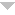orortakenwhy

Make sure to remember your password. If you forget it there is no way for StudyStack to send you a reset link. You would need to create a new account.

Enter the associated with your account, and we'll email you a link to reset your password.

# NPTE Research & EBP

Quiz yourself by thinking what should be in each of the black spaces below before clicking on it to display the answer.
Help

 QuestionAnswerMeasures of central tendency   mean, median, mode
Mean   average of all scores
Median   midpoint of all scores
Mode   most frequently occurring score
mean is appropriate for what data types?   interval, ratio
median is appropriate for what data types?   ordinal data
mode is appropriate for what data types?   nominal
Measures of variability   range, standard deviation, normal distribution, percentiles & quartiles
Range   difference between highest and lowest score
Standard Deviation   variability of scores from the mean. most frequently used
How to calculate SD   subtract each score from mean, square each difference, add up all squares, divide by number of scores
Normal distribution   symmetrical bell shaped curve indiecating distribution of scores. Mean/median/mode all similar.
Inferential statistics   allow determination of how likely results can be generalized to a population
Standard error of measurement   an estimate of expected errors in a score, measure of response stability or reliability
Tests of significance   estimation of true differences not due to chance, rejection of null hypothesis
Alpha level   probability level - reselected level of statistical significance. Most commonly .05 or .01 (.05= only 5x out of 100 is the difference due to chance)
Degrees of freedom   based on # of subjects and groups, allows determination of level of significance
Standard error   result of sampling error, expected chance variation among the means
Type 1 error   Null hypothesis rejected when it is true.
Type 2 error   Null hypothesis is not rejected when it is false. means concluded to be due to chance when truly different
How to decrease type 1/2 errors   increase sample size, random selection, valid measures
Parametric statistics   Interval or Ratio data
Assumptions for parametric statistics   normal distribution (usu large representative samples this is met), random sampling performed, variance in groups is equal
T-test   parametric test of significance used to compare 2 independent groups created by random assignment and ID difference at a selected probability level
T-test for independent samples   compares 2 independent groups
T-test for paired samples   compares 2 matched samples (does therapy incr fxn in siblings with autism)
One-tailed T-test   based on directional hypothesis. Evaluates differences in data on only one end of distribution (neg or pos)
Two-tailed T-test   based on a nondirectional hypothesis. Evaluates differences in data on both ends of a distribution. Tests of signif are almost always two-tailed
Inappropriate use of T-test   use to compare more than 2 means within a single sample.
ANOVA   parametric test used to compare 3 or more independent tx groups at a selected probability level.
Simple (one-way) ANOVA   compares multiple groups on a single IND variable. Ex: Balance Master score for 3 different age groups
Factorial ANOVA   compares multiple groups on two or more IND variables. Ex: 3 levels of ankle injury compared for balance and sensory
ANCOVA   Parametric test used to compare 2 or more treatment groups or conditions while also controlling for the effectss of intervening variables.
Nonparametric statistics   ORDINAL or NOMINAL data, testing not based on population parameters
When to use nonparametric   parametric assumptions cannot be met. used with small sample, ordinal or nominal level data. Less powerful than parametric
Chi square test   nonparametric test of significance. Used to compare data in the form of frequency counts in 2 or more mutually exclusive categories (rate treatment preferences)
Correlational statistics   used to determine the relative strength of a relationship between 2 variables
Pearson product-moment coefficient (r)   used to correlate CONTINUOUS data wi
Linear Regression   used to establish relationship between two variables as a basis of prediction
Spearman's Rank   NONPARAMETRIC test to correlate ORDINAL data.

Embed Code - If you would like this activity on your web page, copy the script below and paste it into your web page.

Normal Size     Small Size show me how
Created by: Jenwithonen